# Unit 4: Lessons 76 – 100

In this lesson, you will learn about a grammatical principle that, by now, you probably hear all the time in Korean. Probably one of the most common grammatical forms in Korean is the usage of ~는데. Let me break this principle down for you in the first two lessons of Unit 4!

This Lesson is also available in EspañolDescription

In the previous lesson, you were introduced to the ~는데 principle. You learned that you can use this grammatical form to express a lighter version of “even though,” while at the same time prepping the scenario for the second clause of the sentence. In this lesson, you will continue to learn about ~는데 (without the space) and ~는 데 (with the space). You will also take a look at the meaning of the often miss-interpreted “그런데.”

This Lesson is also available in EspañolDescription

In the previous two lessons, you were subject to the confusing explanations of the ~는데/~는 데 grammatical principle. Now that those two difficult/confusing lessons are finished, I can go easy on you and describe some grammatical principles that aren’t as difficult to understand. In this lesson, you will learn about ~에 의하다 and ~(으)로 인하다 which, aside from looking very similar, sometimes have similar meanings.

This Lesson is also available in Español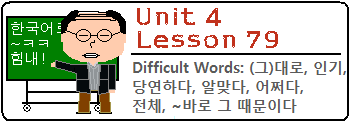Description

Instead of a lesson about some Korean grammatical principles, the vocabulary is the lesson. As with previous “difficult word” lessons, I will teach you some words that cannot be understood by simply looking up the translation in a dictionary. Rather, these words need a detailed description to be fully understood. You will learn about the following words in this lesson: (그)대로, 인기, 당연하다, 알맞다, 어쩌다, 전체, and ~바로 그 때문이다.

This Lesson is also available in Español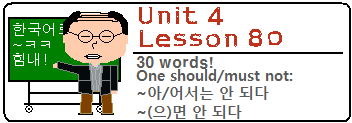Description

In this lesson, you will learn about two similar grammatical principles and their (similar) respective meanings. In this lesson, you will learn how to use ~아/어서는 안 되다 to mean “one should not” and ~(으)면 안 되다 to mean “one must not.” We will also look at the meaning of “되다” as a stand-alone word again, and the positive meaning of using ~(으)면 되다, which is a very natural expression but usually one that foreign learners of Korean cannot understand properly.

This Lesson is also available in EspañolDescription

In this lesson, you will learn how to use ~(으)니까 and ~(으)니between two clauses to create a meaning that is similar to ~아/어서. Of course, just like every other grammatical principle, there are some subtle nuances that you should know about.

This Lesson is also available in Español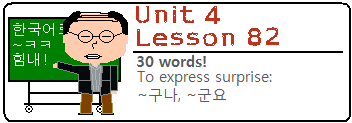Description

In this lesson you will learn how to add ~구나, ~군 or ~군요 to the end of a sentence.  This grammatical form is said when somebody realizes a new fact or piece of information.

This Lesson is also available in Español

In this lesson, you will learn the meaning of adding ~네(요) to sentences. This grammatical form can be used to express surprise, and has a very similar meaning to ~구나/~군/~군요, which you learned in the previous lesson.

This Lesson is also available in Español

________________________
Lessons 75 – 83 Mini Test
________________________

In this lesson, you will learn about the common grammatical principle ~자마자 and its less common counterpart, ~자. Both have similar meanings, but as always I will dissect each one so you can understand them more clearly by the end of this lesson.

This Lesson is also available in Español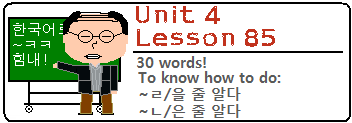Description

In this lesson, you will learn two grammatical principles that can be used with the verb 알다 (to know). First, you will learn about ~ㄹ/을 줄 알다 [/모르다] (to know how) and second you will learn about 알다시피 (as you know).

This Lesson is also available in EspañolDescription

In this lesson, you will learn how to use the word 아니라 (from the word 아니다) to negate a preceding noun. In addition, you will also learn how this same grammatical principle can negate an entire clause ending in an adjective or verb in the form of ~는 게 아니라.

This Lesson is also available in EspañolDescription

In this lesson, you will learn how to add ~기로 하다 to the end of a sentence or clause to have the meaning “to decide to.” The usage and translation are fairly straightforward, to the point that the construction can almost be thought of as an idiom. However, I do feel that this is a grammatical principle that deserves its own lesson.

This Lesson is also available in Español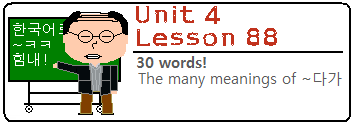Description

In this lesson, you will learn about the various usages of ~다가, which is often placed between two clauses. The difficulty with ~다가 is that there are many meanings that this grammatical principle can take on – all with a seemingly identical purposes. Because of this confusion, this grammatical principle has plagued learners of Korean for years.

This Lesson is also available in Español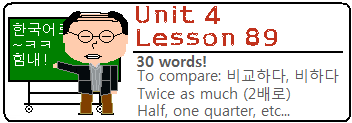Description

In this lesson, you will learn a variety of new words and grammatical principles that you can use in comparative sentences. You will learn about 비교하다 and ~에 비해 and how to use fractions and how to say things like “two times better” or “half as good,” which are commonly used in comparison sentences.

This Lesson is also available in EspañolDescription

In this lesson you will learn how to end sentences with ~잖다 (~잖아 or ~잖아요). Though this is a very common ending in conversational Korean, English speakers often cannot figure out exactly what it means. The main reason for this is simply because it is hard to translate directly into English. In this lesson, there will be many examples that describe specifically the situation where this grammatical form can be used.

This Lesson is also available in Español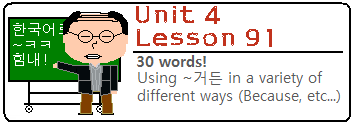Description

In this lesson, you will learn how to add ~거든(요) to the end of a sentence or to connect two clauses. Though this grammatical form has one very common meaning, it is often used to have other meanings as well. In this lesson, I will break down all the meanings of ~거든(요) and explain how they can be used.

This Lesson is also available in Español

________________________
Lessons 84 – 91 Mini Test
________________________

In this lesson, you will learn about the Korean grammatical principle of ~도록, which has confused foreign learners of Korean for ages. There are three common meanings of ~도록, but I will break it all down for you step by step, so you have no reason to worry.

In this lesson, you will learn how to add ~지 or its formal equivalent ~죠 to the end of a sentence. The meaning that this grammatical form has is usually tricky for foreign learners of Korean to pick up on – but don’t worry, I am here to explain everything to you!

In this lesson, you will learn about adding ~게 되다 to verbs or adjectives. In Lesson 14 you learned about 되다 and how it is used in passive sentences. Using 되다 in the ~게 되다 has a different meaning that needs to be discussed in this lesson.

In this lesson, you will learn how to use 뿐 in a variety of situations. First, you will learn how to use 뿐 after a noun. Then, you will learn how to use 뿐 after a verb/adjective – both at the end of a sentence and in the middle of a sentence. Finally, you will learn how to use ~뿐만 아니라 to have the meaning of “not only A, but B.”

In this lesson, you will learn how to connect two clauses with ~(으)려면 to have the meaning of “if one wants to be able to” or “if one intends to.”

In this lesson, you will learn about the word 갖다, which is actually a colloquial abbreviation of the word “가지다.” The Korean language has evolved in such a way that “갖다” can now be thought of as a word, but due to the fact that it is actually an abbreviation, it has atypical grammatical rules. I will break these rules open for you.

In this lesson, you will learn about a grammatical principles that is essentially an extension of the ~는 것 principle that you studied at great lengths in the first five lessons of Unit 2. 척(하다) can be used instead of “것” in the “~는 것” principle to mean “to pretend.”

In this lesson, you will learn how to use the ~더라도 as a connector between two clauses to mean “even if.” Though this form sometimes may look similar to “Even though” (~지만) or “If” (~ㄴ/는다면), I will distinguish them for you in this lesson.

Our 100th lesson! In this lesson, you will learn about the grammatical principle ~ㄹ/을 텐데(요), and to a lesser extend ~ㄹ/을 테니까.

________________________

Lessons 92 – 100 Mini Test
________________________

Unit 4 Test
________________________